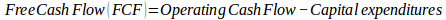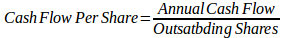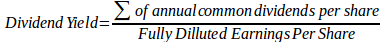# The Easiest Ways to Calculate Dividend Yield

##Knowing how to find debt to equity ratio and how to find dividend yield is very important in accounting formulas since dividends account for almost a third of total returns on equity in stock markets. Therefore, investors solely focus on earnings provided by payment of dividends. Stock appreciation or what is commonly referred to as dividends are the returns investors receive when they invest money in stock.

Income stocks are the shares most companies pay to investors to offset investors’ interest, and growth stocks are shares which organizations choose to reinvest in the business leaving investors with no dividends. Financial ratios are classified according to the business’ financial feature which they measure. Dividend yield ratio is expressed as a percentage and indicates the shares a company pays out on an annual basis relative to its share price. Investors calculate dividend yield to analyze their investment returns in stocks. They show whether their investment in stock is generating stock appreciation through increases in assets or dividends through cash flows.Image credit: WikiHow

Cautious investors should focus on dividend yield since it states the extent to which organizations can manage payouts at the current dividend level. The dividend yield ratio formula is:Below, we provide a step-by-step procedure for how is dividend yield calculated in three ways.

## Calculating Dividend Yield as a Percentage of Free Cash Flow

This payout ratio is conservative since the capital expenditure value varies because some companies invest heavily as compared to others.

• Find the Free Cash Flow (FCF) figure by subtracting capital expenditures of the previous financial year from operating cash flow. Capital expenditures are present in the investment activities part of the cash flow statement. Assume the operating cash flow is \$100 million and capital expenditures \$40 million.FCF = \$100 million – \$30 million

= \$70 million

• Obtain the total dividends value from the financing activities part. Our company’s total dividend value is \$60 million.
• Divide total dividends with FCF to get the company’s dividend yield.= \$60 million/ \$70 million

= 0.86

## Calculating the Dividend Yield as a Percentage of Operating Cash Flow

Obtaining the dividend yield as a percentage of cash flow from operations is derived by summing up net income and non-cash charges which are a more accurate and less conservative method of determining a company’s dividend sustainability. Some people refer to non-cash charges such as amortization and depreciation as earnings per share. Here are the steps involved:

• Find the annual operating cash flow value from the previous year’s statement of cash flow. For example, our company has \$100 million fully diluted outstanding shares and an annual cash flow of \$100 million. The cash flow per share value is derived by dividing annual cash flow and outstanding shares.= \$100 million/\$100 million

In our case, the cash flow per share is \$1 per share.

• Obtain the common dividends value of the same year. Our company’s common dividends amount to \$0.70
• Get the dividend yield.= \$0.70/\$1

= 0.7

## Calculating Dividend Yield as a Percentage of Earnings

The general dividend yield formula is used in calculating the indicated dividend payout ratio and current dividend payout ratio. The two ratios vary in companies that regularly increase dividends annually while they are similar for companies with a constant dividend level every year.

The process of calculating dividend yield as calculating percents of earnings involves:

• Obtain the summation of the company’s earnings per share for the previous four quarters which is not similar to the EPS value found in the Statement of Comprehensive Income. The summed up value is known as the Fully-Diluted Earnings per Share (FDEPS). Let our company’s EPS in the last four quarters be \$0.25, \$0.20, \$0.15, and \$0.30 for Q1, Q2, Q3, and Q4 respectively. `The FDEPS will be 0.9
• Obtain the sum of common dividends per share of the previous year. Assume our company’s dividend per share was \$0.15 for the first quarter. The value of dividends per share for the previous year will be) \$0.6.
• Find the dividend yield.= \$0.6/\$0.9

=0.54

## What Is a Good Dividend Yield?

According to dividend.com, a good dividend yield ranges from 0 to 0.35. It is usually for companies that require almost all their cash to facilitate business growth. For example, technology companies have a desire to develop and conduct intensive research, hence the need to retain almost all their earnings. A healthy dividend yield ranges from 0.35 to 0.55. Companies that have the ability to offer more than half of their earnings as dividends implies that they are a leader in the industry and well established. High dividends ranging from 0.55 to 0.95 are good for the investors but dangerous for the company. A high dividend yield means that a company has less retained earnings for growth and giving dividends in the future due to their inability to utilize capital expenditure. Unsustainable dividend yields exceed 0.95 which is risky since it implies that a company is distributing dividends and incurring debt. Investors should choose healthy dividend yields over high dividend yields since the former is likely to be long-term as compared to the latter.

As you’ve seen, there is a lot involved in these calculations, and sometimes students tend to make mistakes or mix up formulas when they carry them out. Besides, if you are not familiar with these processes, you tend to take longer than usual to get the assignment done, and sometimes you end up failing. Finance Homework Help is a group of experienced professional finance specialists who know these kinds of things inside out.

We show you how to master accounting formulas, how to get average dividend yield, how to construct finance formula sheet, and how to conduct ratio analysis such as how to find debt-to-equity-ratio. We work out your finance assignments at affordable prices and at the same time guarantee on time delivery, free adjustments, comprehensive explanations, and precise calculations. You can call us for free, post queries on our ever live chat tool, send us an email, and you can even choose your own tutor!